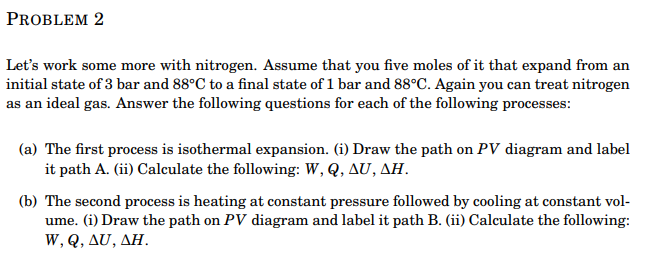# Question Solved1 Answer PROBLEM 2 Let's work some more with nitrogen. Assume that you five moles of it that expand from an initial state of 3 bar and 88°C to a final state of 1 bar and 88°C. Again you can treat nitrogen as an ideal gas. Answer the following questions for each of the following processes: (a) The first process is isothermal expansion. (i) Draw the path on PV diagram PROBLEM 2 Let's work some more with nitrogen. Assume that you five moles of it that expand from an initial state of 3 bar and 88°C to a final state of 1 bar and 88°C. Again you can treat nitrogen as an ideal gas. Answer the following questions for each of the following processes: (a) The first process is isothermal expansion. (i) Draw the path on PV diagram and label it path A. (ii) Calculate the following: W, Q, AU, AH. (b) The second process is heating at constant pressure followed by cooling at constant vol- ume. (i) Draw the path on PV diagram and label it path B. (ii) Calculate the following: W, Q, ΔU, ΔΗ.YBMGKM The Asker · Chemical EngineeringTranscribed Image Text: PROBLEM 2 Let's work some more with nitrogen. Assume that you five moles of it that expand from an initial state of 3 bar and 88°C to a final state of 1 bar and 88°C. Again you can treat nitrogen as an ideal gas. Answer the following questions for each of the following processes: (a) The first process is isothermal expansion. (i) Draw the path on PV diagram and label it path A. (ii) Calculate the following: W, Q, AU, AH. (b) The second process is heating at constant pressure followed by cooling at constant vol- ume. (i) Draw the path on PV diagram and label it path B. (ii) Calculate the following: W, Q, ΔU, ΔΗ.
More
Transcribed Image Text: PROBLEM 2 Let's work some more with nitrogen. Assume that you five moles of it that expand from an initial state of 3 bar and 88°C to a final state of 1 bar and 88°C. Again you can treat nitrogen as an ideal gas. Answer the following questions for each of the following processes: (a) The first process is isothermal expansion. (i) Draw the path on PV diagram and label it path A. (ii) Calculate the following: W, Q, AU, AH. (b) The second process is heating at constant pressure followed by cooling at constant vol- ume. (i) Draw the path on PV diagram and label it path B. (ii) Calculate the following: W, Q, ΔU, ΔΗ.